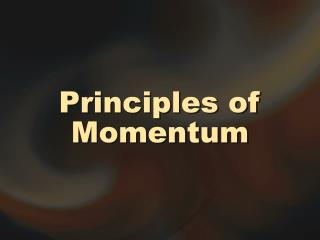DownloadDownload PresentationPrinciples of Momentum

# Principles of Momentum

Télécharger la présentation## Principles of Momentum

- - - - - - - - - - - - - - - - - - - - - - - - - - - E N D - - - - - - - - - - - - - - - - - - - - - - - - - - -
##### Presentation Transcript

1. Principles of Momentum

2. Momentum • defined: a measure of an object’s inertia of motion • What physical properties affect momentum? • mass • velocity

3. Momentum • defined mathematically: p = mv • units: kg·m/s • Linear momentum is a vector in the same direction as the velocity vector.

4. Δp ΣF= Δt Momentum • since the object’s mass is usually constant: Δp = mΔv • after doing some algebra:

5. Δp ΣF= Δt Momentum • Momentum results from an unbalanced force. • This is how Newton originally stated his second law of motion.

6. Impulse • The longer an unbalanced force is applied, the greater its change in momentum. • defined: the product of a force and the time the force is exerted

7. Impulse • defined mathematically: I = ΣFΔt or I = Δp impulse vector has same magnitude and direction as Δp vector

8. Impulse • “Impulsive force” generally refers to forces on a system that are large in comparison to the other forces. • Example 11-2 shows the importance of time to a change of momentum.

9. Conservation of Momentum • When no unbalanced external forces act on a system, the momentum of a system stays the same. • The recoil of a firearm demonstrates conservation of momentum.

10. Conservation of Momentum • The mass can vary, such as in a space vehicle. • The change in momentum of the rocket has equal magnitude and opposite direction as the momentum of the propellant exhaust.

11. ( ) Δmprop Fexh x = -vexh x = mrar x Δt Conservation of Momentum • Thrust: Fexh x Fexh x = mrar x

12. Collisions

13. Linear Collisions • gliding masses on an air track—momentum is conserved ptotalbfr = p1 bfr + p2 bfr ptotal aft = p1 aft + p2 aft Δp1 = -Δp2

14. Elastic Collisions • only conservative forces act • total mechanical energy conserved

15. Partially Elastic Collisions • one or more colliding masses is deformed • total mechanical energy not conserved • some kinetic energy converted to other forms

16. Inelastic Collisions • colliding masses stick together • kinetic energy is not conserved

17. m1 - m2 ( ) v1 aft x = v1 bfr x m1 + m2 ( ) 2m1 v2 aft x = v1 bfr x m1 + m2 Elastic Collisions • kinetic energy is conserved • two important equations:

18. Elastic Collisions • equations are for an elastic collision where a moving m1 collides with a resting m2 • if v1 aft x is negative then m1 has rebounded in the opposite direction from which it came

19. Elastic Collisions • Example 11-4: equal masses on an air track • Example 11-5: large object collides with small object • Example 11-6: small object collides with large object

20. Inelastic Collisions • only one equation needed: m1 v1 bfr x = (m1 + m2) vaft x • Example 11-7 illustrates that kinetic energy is not conserved in such collisions.

21. Ballistic Pendulums • used to determine the energy of projectiles such as bullets • kinetic energy is almost completely converted to potential energy in the pendulum

22. Two-Dimensional Collisions • Most collisions occur in two (or three) dimensions. • Air tables are often used to illustrate these. • Δp1 = -Δp2 is still true.

23. Two-Dimensional Collisions • Since momentum is still conserved... • x-component of the momentum before the collision must equal the sum of the x-components afterward • also true of y-components

24. Two-Dimensional Collisions • When two particle-like objects collide elastically, the post-collision angle between the object’s paths is exactly 90°. • The angle decreases as the deformation in the objects increases.

25. Center of Mass and Angular Momentum

26. Center of Mass • defined: the point in space where the mass of the entire system is assumed to be located for the purpose of determining the momentum of the system

27. Center of Mass • in the explosion example: psysbfr = Σpi aft • Σpi is the vector sum of the momenta of all of the individual particles. • momentum is conserved • total kinetic energy is not conserved

28. Angular Momentum • A mass moving in a circle has momentum associated with its circular motion. • For a single orbiting particle: L = mr²ω

29. Angular Momentum • L = angular momentum • m = mass • r = radius of circular path • ω = angular speed L = mr²ω

30. Angular Momentum • If the mass stays constant and the radius reduces to half, then the angular speed quadruples. L = mr²ω

31. Angular Momentum • is a vector quantity • direction is found using the rotational right-hand rule